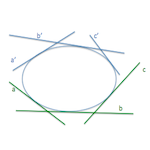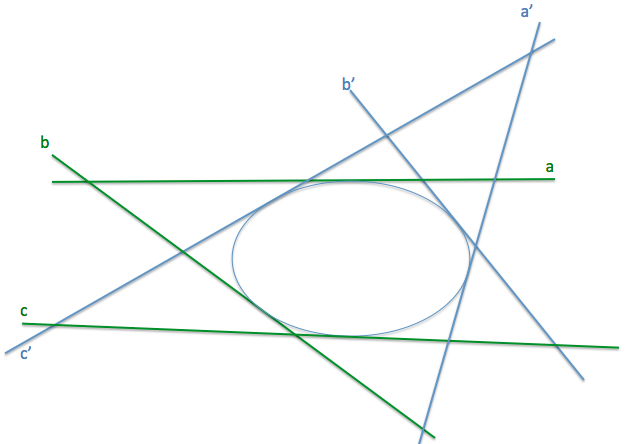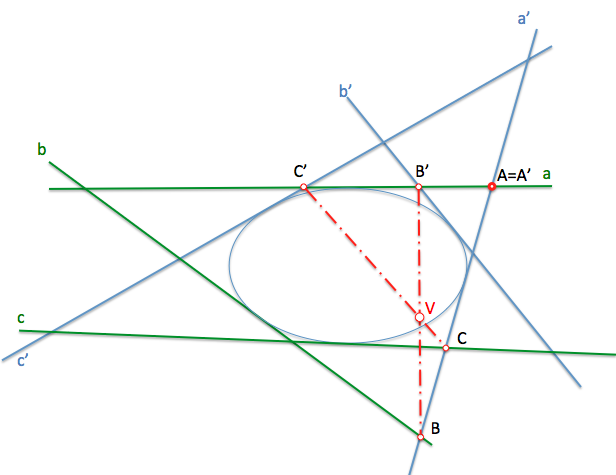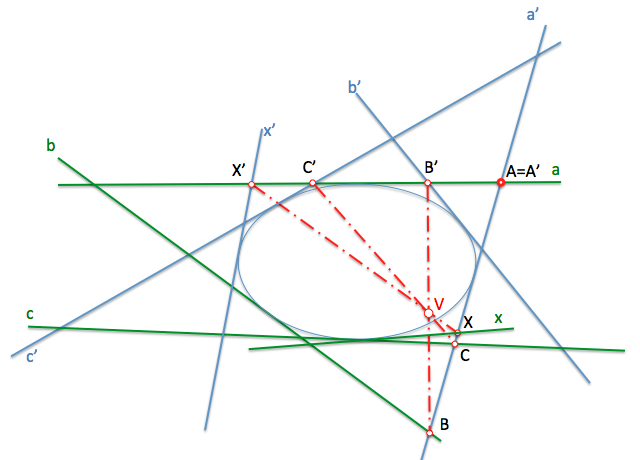# Geometría proyectiva: You do overlapping of second orderWe have seen the projective generation of the Conic from two dual approaches:

Conical point: The taper is determined by the endless points of intersection of two projective bundles

Conical tangential: The taper is determined by the endless lines that projected homologous elements of two projective series.

To study the tangential Conic, and in particular the proyectividades between beams of second order superimposed on a same curve, We can rely on the dual study carried out with the overlapping series of second order.

Do the work with these procedure is analogous to which he saw to the get items counterparts in the proyectividades between two beams of the first order, in which we determined intermediate outlook forms (series of points) determining its Center perspective that we called"projective center beams".

The is between two beams overlapping second order will be determined when we know three pairs of tangent counterparts on the same conical. (a-a’, b-b’, c-c’)

Recall that a cone will be determined by five conditions (the tangent points straight). As additional comments, Remember that a straight line is determined by two of your points, But if we want to define an is between overlapping series we need to relate three pairs of points that belong to the straight.

In Figure, the is is defined by pairs of homologous straight a-a. ', b-b' and c '.If we dissect by two straight counterparts (for example to and a’) the elements of each beam are obtained prospects series since they have a double point (A-A’). These series will be screened from its Center perspective that will be the "projective Center of bundles of second order". This point, V in Figure, "is known by the nickname of"Brianchon point"To determine the homologous straight x element either operate as with the beams of the first order. We then the straight x by an element (the ') to obtain the X point in previous perspectives series. The point of the homologous series, X’, you will be aligned with the center of series perspective (projective center beams) and will contain the straight x' counterpart from x.Tangents from the projective Center, If they exist, determine the dual elements of the overlapping beams of second order. To check this, We will obtain the counterpart of these rays considering them belonging to any of the beams, as we have done with previously transformed x ray. The reader is left checking.

Note that this analysis is shown tapered to improve understanding of the concepts. As the conical not we generally, obtaining the element x' counterpart of x must be carried out by earning two points, repeating the procedure in section with a new tangent.

Projective Geometry